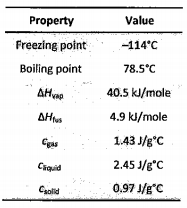# Problem: From the data below, calculate the heat (kJ) involved in the transformation of 0.450 mol of ethanol (CH3CH2OH) gas at 275°C and 1 atm to solid ethanol at -116°C.

###### FREE Expert Solution

We’re being asked to calculate the amount of energy released when 0.450 moles of ethanol gas is cooled from 275 ˚C to -116.0 ˚C (solid ethanol).

Note that cooling and freezing is an exothermic process, which means q is negative (–)

There are two heats involved in this problem:

1. q1 which is the heat in cooling 0.450 moles of gaseous ethanol from 275 ˚C to 78.5 ˚C

2. q2 which is the heat in condensing 0.450 moles of H2O at 78.5 ˚C

3. q3 which is the heat in cooling 0.450 moles of liquid ethanol from 78.5 ˚C to -114˚C

4. q3 which is the heat in freezing 0.450 moles of H2O at -114˚C

5. q3 which is the heat in cooling 0.450 moles of solid ethanol from -114˚C to -116 ˚C

We need to solve for each heat individually then add them together to get the final answer.###### Problem Details

From the data below, calculate the heat (kJ) involved in the transformation of 0.450 mol of ethanol (CH3CH2OH) gas at 275°C and 1 atm to solid ethanol at -116°C.